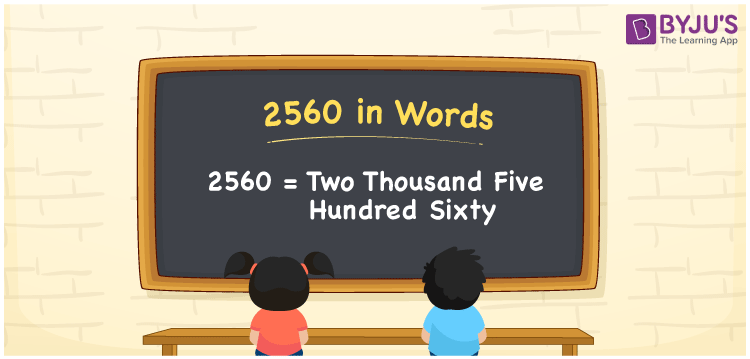# 2560 in Words

2560 in words is written as Two thousand five hundred sixty. In both the International System of Numerals and the Indian System of Numerals, 2560 is written as Two thousand five hundred sixty. The number 2560 is a Cardinal Number as it represents some quantity. For example, “that item costs 2560 rupees”.

 2560 in Words Two thousand five hundred sixty Two thousand five hundred sixty in Number 2560

## 2560 in English Words

2560 in English words is read as “Two thousand five hundred sixty”.## How to Write 2560 in Words?

To write 2560 in words, we shall use the place value chart. In the place value chart, put 2 in the thousands, 5 in the hundreds, 6 in the tens, and 0 in the ones, respectively. Let us make a place value chart to write the number 2560 in words.

 Thousands Hundreds Tens Ones 2 5 6 0

Thus, we can write the expanded form as

2 × Thousand + 5 × Hundred + 6 × Ten + 0 × One

= 2 × 1000 + 5 × 100 + 6 × 10 + 0 × 1

= 2000 + 500 + 60 + 0

= 2560

= Two thousand five hundred sixty.

2560 is a natural number, the successor of 2559 and the predecessor of 2561.

2560 in words – Two thousand five hundred sixty

• Is 2560 an odd number? – No
• Is 2560 an even number? – Yes
• Is 2560 a perfect square number? – No
• Is 2560 a perfect cube number? – No
• Is 2560 a prime number? – No
• Is 2560 a composite number? – Yes

## Frequently Asked Questions on 2560 in Words

Q1

### How to write 2560 in words?

2560 in words is written as Two thousand five hundred sixty.
Q2

### How to write 2560 in words in the International and Indian System of Numerals?

In both, the system of numerals, 2560 in words, is written as Two thousand five hundred sixty.
Q3

### How to write 2560 in a place value chart?

In the place value chart, write 2 in the thousands, 5 in the hundreds, 6 in the tens, and 0 in the ones, respectively.